## Synopsis

This objective of this analysis and modelling is to review time series theory and experiment with R packages.

We will be following an ARIMA modeling procedure of the AirPassengers dataset as follows:
1. Perform exploratory data analysis
2. Decomposition of data
3. Test the stationarity
4. Fit a model used an automated algorithm
5. Calculate forecasts

## Setup

library(ggfortify)
## Loading required package: ggplot2
library(tseries)
library(forecast)
##
## Attaching package: 'forecast'
## The following object is masked from 'package:ggfortify':
##
##     gglagplot

data(AirPassengers)
AP <- AirPassengers
# Take a look at the class of the dataset AirPassengers
class(AP)
##  "ts"

The AirPassenger dataset in R provides monthly totals of a US airline passengers, from 1949 to 1960. This dataset is already of a time series class therefore no further class or date manipulation is required.

## Part 1: PERFORM EXPLORATORY DATA ANALYSIS

To perform exploratory analysis, let’s first review the data with summary statistics and plots in R.

# Take a look at the entries
AP
##      Jan Feb Mar Apr May Jun Jul Aug Sep Oct Nov Dec
## 1949 112 118 132 129 121 135 148 148 136 119 104 118
## 1950 115 126 141 135 125 149 170 170 158 133 114 140
## 1951 145 150 178 163 172 178 199 199 184 162 146 166
## 1952 171 180 193 181 183 218 230 242 209 191 172 194
## 1953 196 196 236 235 229 243 264 272 237 211 180 201
## 1954 204 188 235 227 234 264 302 293 259 229 203 229
## 1955 242 233 267 269 270 315 364 347 312 274 237 278
## 1956 284 277 317 313 318 374 413 405 355 306 271 306
## 1957 315 301 356 348 355 422 465 467 404 347 305 336
## 1958 340 318 362 348 363 435 491 505 404 359 310 337
## 1959 360 342 406 396 420 472 548 559 463 407 362 405
## 1960 417 391 419 461 472 535 622 606 508 461 390 432
# Check for missing values
sum(is.na(AP))
##  0
# Check the frequency of the time series
frequency(AP)
##  12
# Check the cycle of the time series
cycle(AP)
##      Jan Feb Mar Apr May Jun Jul Aug Sep Oct Nov Dec
## 1949   1   2   3   4   5   6   7   8   9  10  11  12
## 1950   1   2   3   4   5   6   7   8   9  10  11  12
## 1951   1   2   3   4   5   6   7   8   9  10  11  12
## 1952   1   2   3   4   5   6   7   8   9  10  11  12
## 1953   1   2   3   4   5   6   7   8   9  10  11  12
## 1954   1   2   3   4   5   6   7   8   9  10  11  12
## 1955   1   2   3   4   5   6   7   8   9  10  11  12
## 1956   1   2   3   4   5   6   7   8   9  10  11  12
## 1957   1   2   3   4   5   6   7   8   9  10  11  12
## 1958   1   2   3   4   5   6   7   8   9  10  11  12
## 1959   1   2   3   4   5   6   7   8   9  10  11  12
## 1960   1   2   3   4   5   6   7   8   9  10  11  12
# Review the table summary
summary(AP)
##    Min. 1st Qu.  Median    Mean 3rd Qu.    Max.
##   104.0   180.0   265.5   280.3   360.5   622.0
# Plot the raw data using the base plot function
plot(AP,xlab="Date", ylab = "Passenger numbers (1000's)",main="Air Passenger numbers from 1949 to 1961")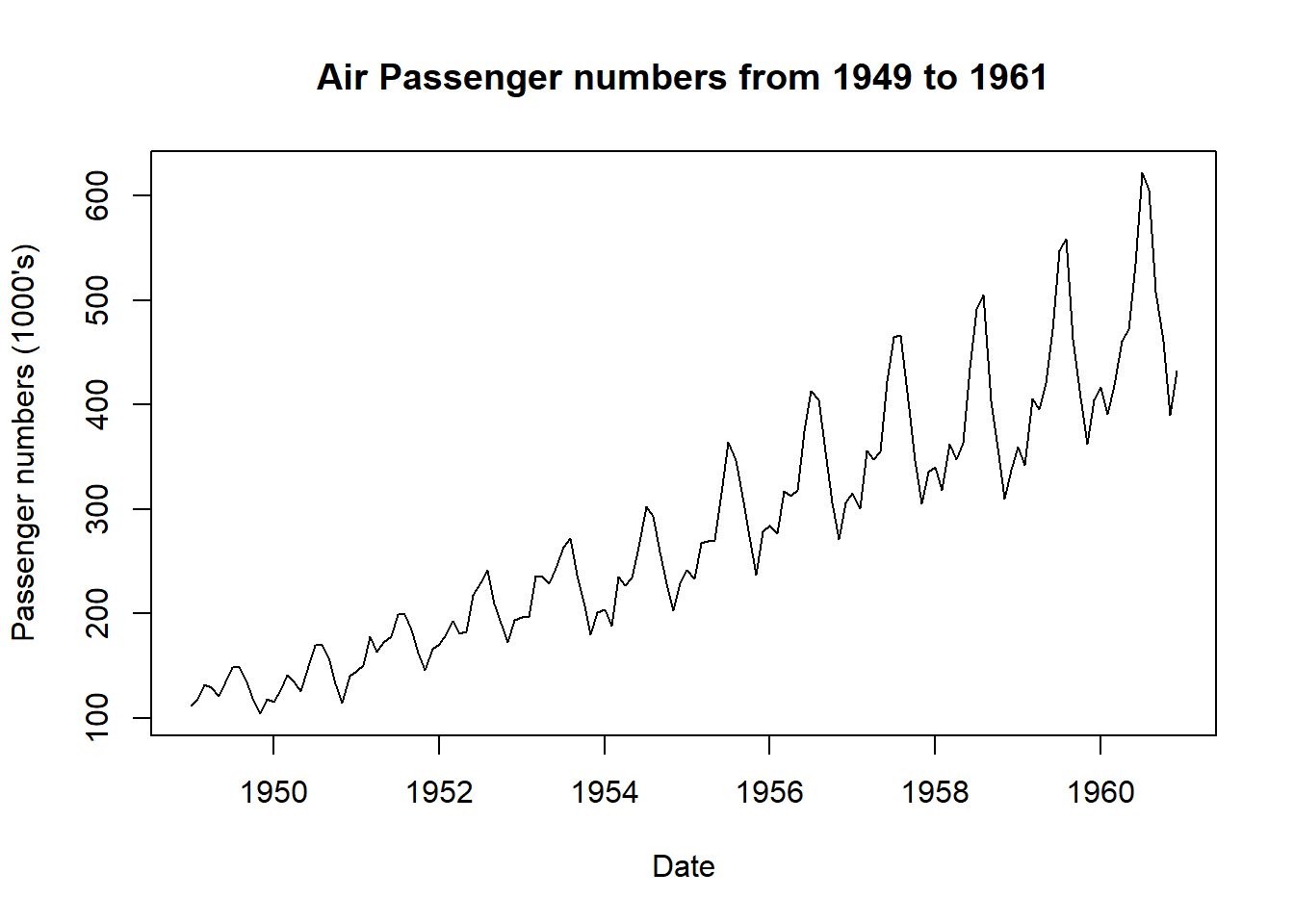As an alternative to the base plot function, so we can also use the extension ggfortify R package from the ggplot2 package, to plot directly from a time series. The benefits are not having to convert to a dataframe as required with ggplot2, but still having access to the layering grammar of graphics.

autoplot(AP) + labs(x ="Date", y = "Passenger numbers (1000's)", title="Air Passengers from 1949 to 1961")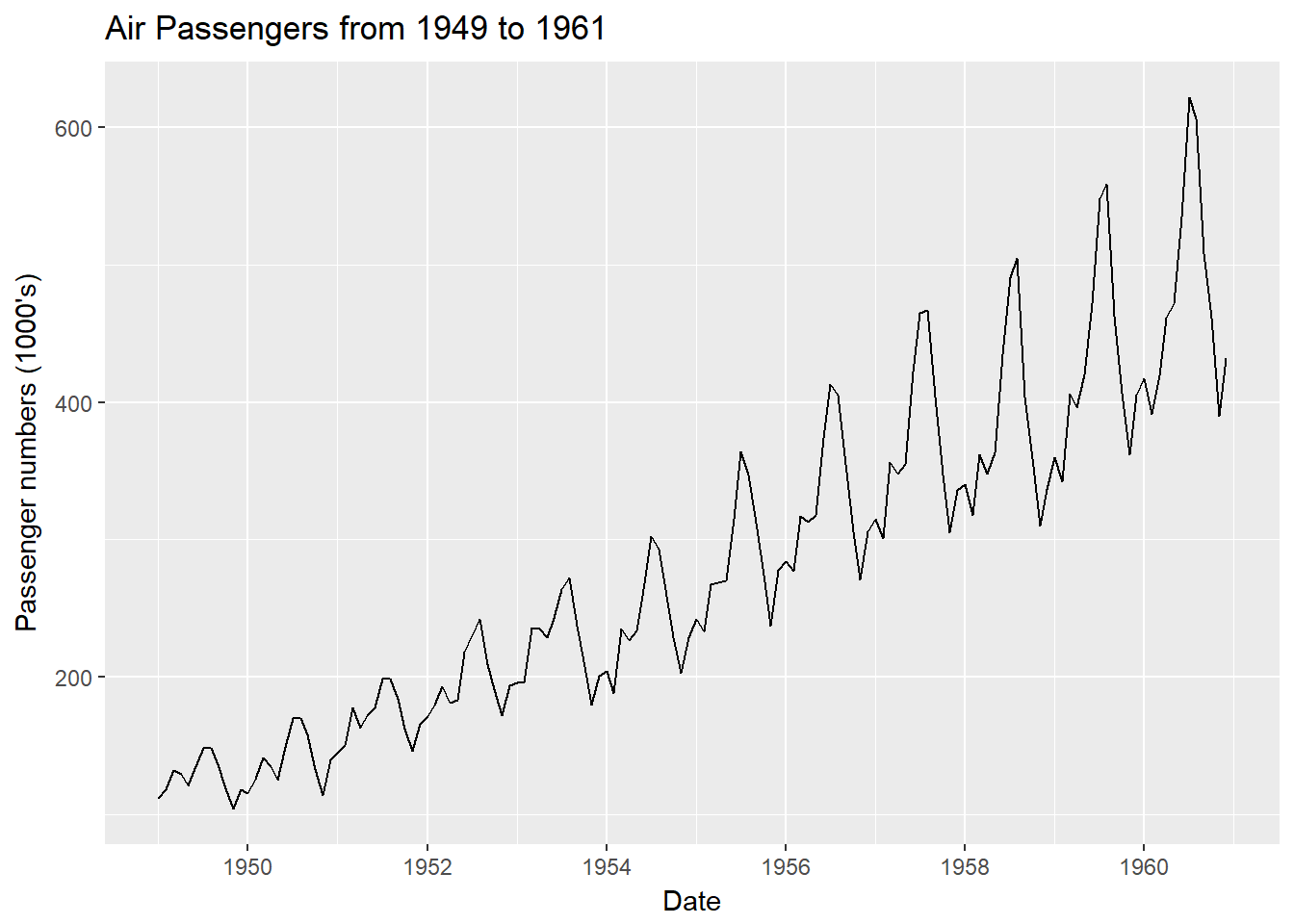Let’s use the boxplot function to see any seasonal effects.

boxplot(AP~cycle(AP),xlab="Date", ylab = "Passenger Numbers (1000's)" ,main ="Monthly Air Passengers Boxplot from 1949 to 1961")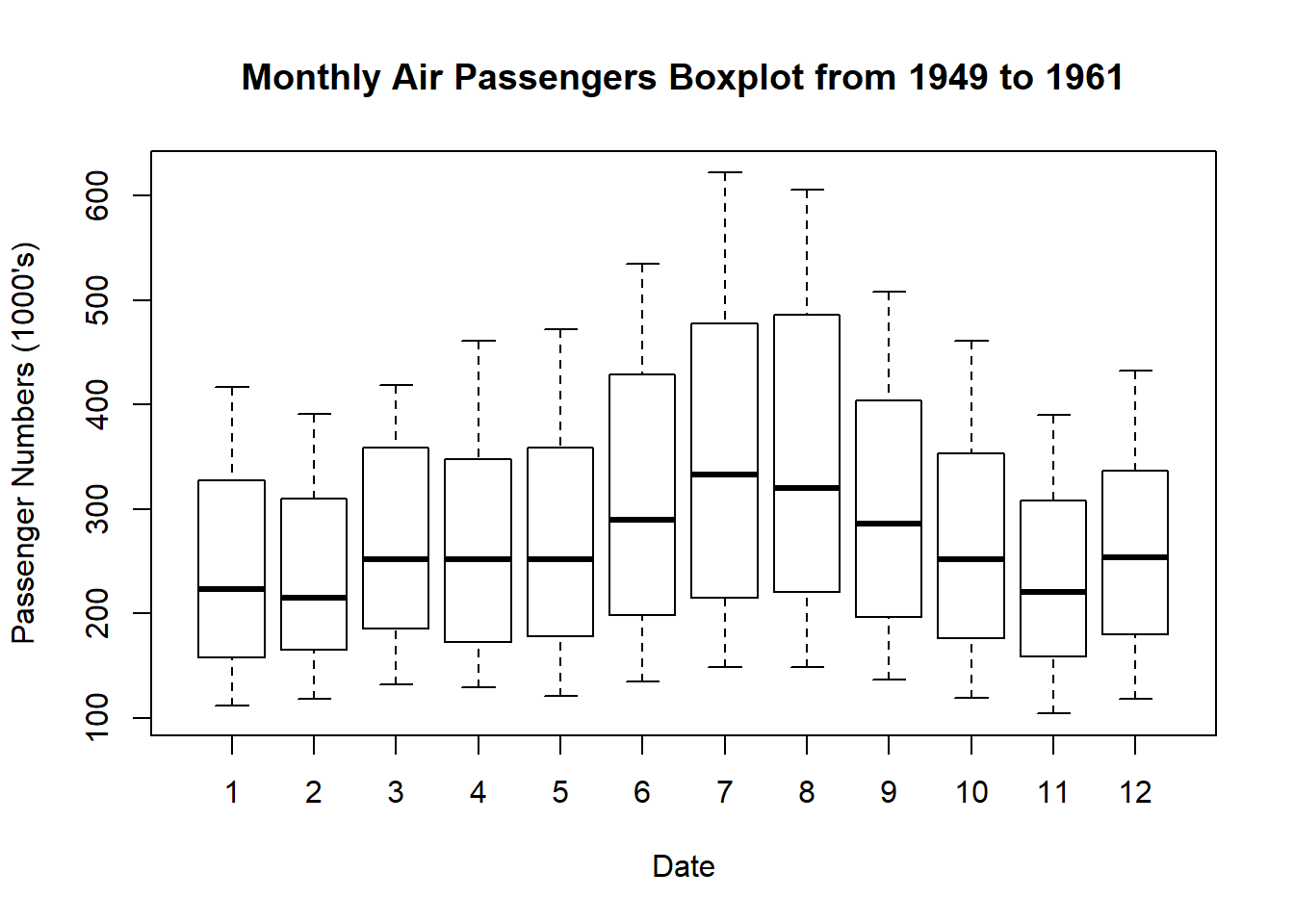From these exploratory plots, we can make some initial inferences:

• The passenger numbers increase over time with each year which may be indicative of an increasing linear trend, perhaps due to increasing demand for flight travel and commercialisation of airlines in that time period.
• In the boxplot there are more passengers travelling in months 6 to 9 with higher means and higher variances than the other months, indicating seasonality with a apparent cycle of 12 months. The rationale for this could be more people taking holidays and fly over the summer months in the US.
• AirPassengers appears to be multiplicative time series as the passenger numbers increase, it appears so does the pattern of seasonality.
• There do not appear to be any outliers and there are no missing values. Therefore no data cleaning is required.

## Part 2: TIME SERIES DECOMPOSITION

We will decompose the time series for estimates of trend, seasonal, and random components using moving average method.

The multiplicative model is:

$$Y[t]=T[t]*S[t]*e[t]$$

where

• Y(t) is the number of passengers at time t,
• T(t) is the trend component at time t,
• S(t) is the seasonal component at time t,
• e(t) is the random error component at time t.

With this model, we will use the decompose function in R. Continuing to use ggfortify for plots, in one line, autoplot these decomposed components to further analyse the data.

decomposeAP <- decompose(AP,"multiplicative")
autoplot(decomposeAP)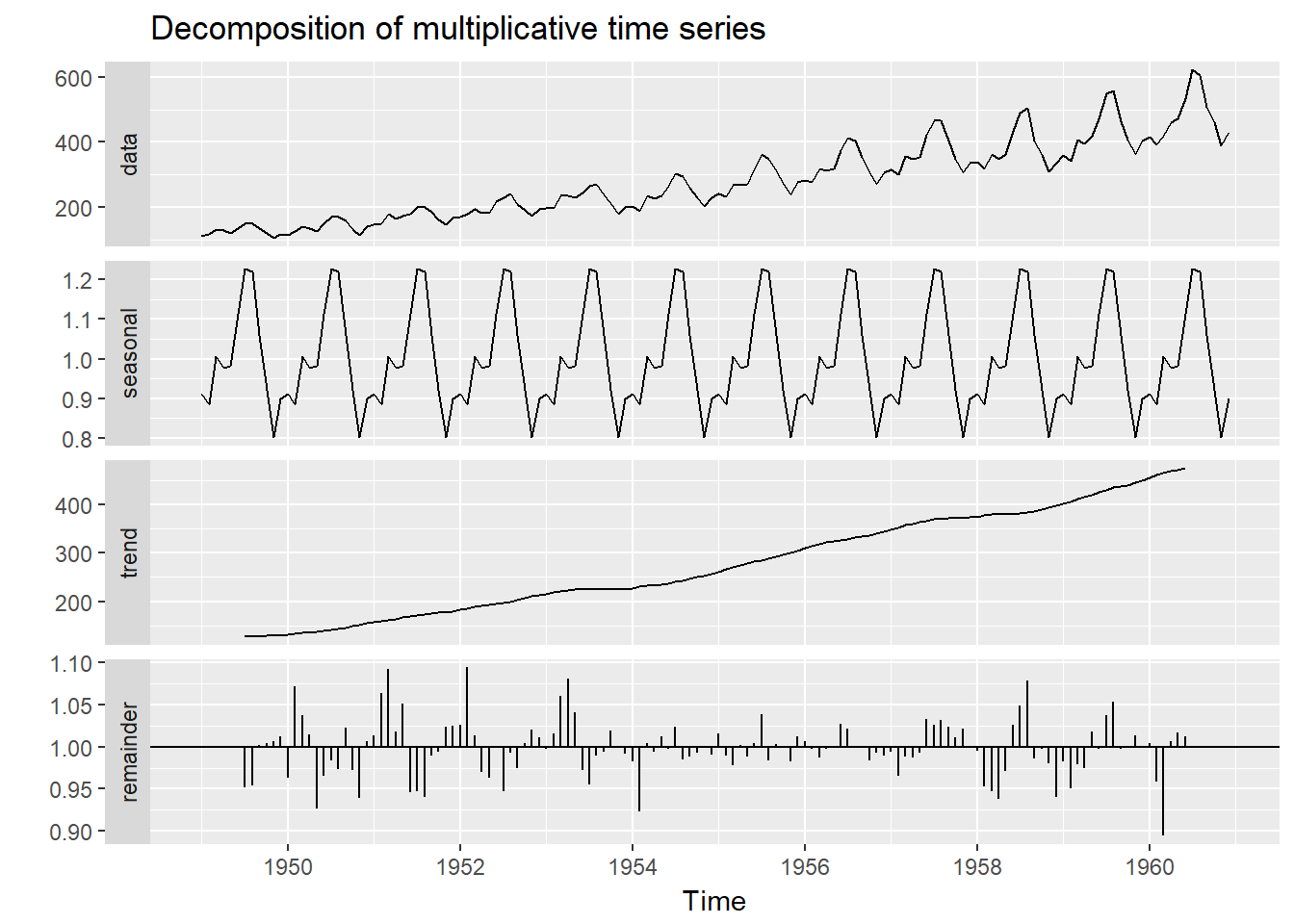In these decomposed plots we can again see the trend and seasonality as inferred previously, but we can also observe the estimation of the random component depicted under the “remainder”.

## Part 3: TEST STATIONARITY OF THE TIME SERIES

A stationary time series has the conditions that the mean, variance and covariance are not functions of time. In order to fit arima models, the time series is required to be stationary. We will use two methods to test the stationarity.

1. Test stationarity of the time series (ADF)

In order to test the stationarity of the time series, let’s run the Augmented Dickey-Fuller Test using the adf.test function from the tseries R package.

First set the hypothesis test:

The null hypothesis $$H_0$$ : that the time series is non stationary
The alternative hypothesis $$H_A$$ : that the time series is stationary

adf.test(AP) 
##
##  Augmented Dickey-Fuller Test
##
## data:  AP
## Dickey-Fuller = -7.3186, Lag order = 5, p-value = 0.01
## alternative hypothesis: stationary

As a rule of thumb, where the p-value is less than 5%, we strong evidence against the null hypothesis, so we reject the null hypothesis. As per the test results above, the p-value is 0.01 which is <0.05 therefore we reject the null in favour of the alternative hypothesis that the time series is stationary.

2. Test stationarity of the time series (Autocorrelation)

Another way to test for stationarity is to use autocorrelation. We will use autocorrelation function (acf) in from the base stats R package. This function plots the correlation between a series and its lags ie previous observations with a 95% confidence interval in blue. If the autocorrelation crosses the dashed blue line, it means that specific lag is significantly correlated with current series.

autoplot(acf(AP,plot=FALSE))+ labs(title="Correlogram of Air Passengers from 1949 to 1961")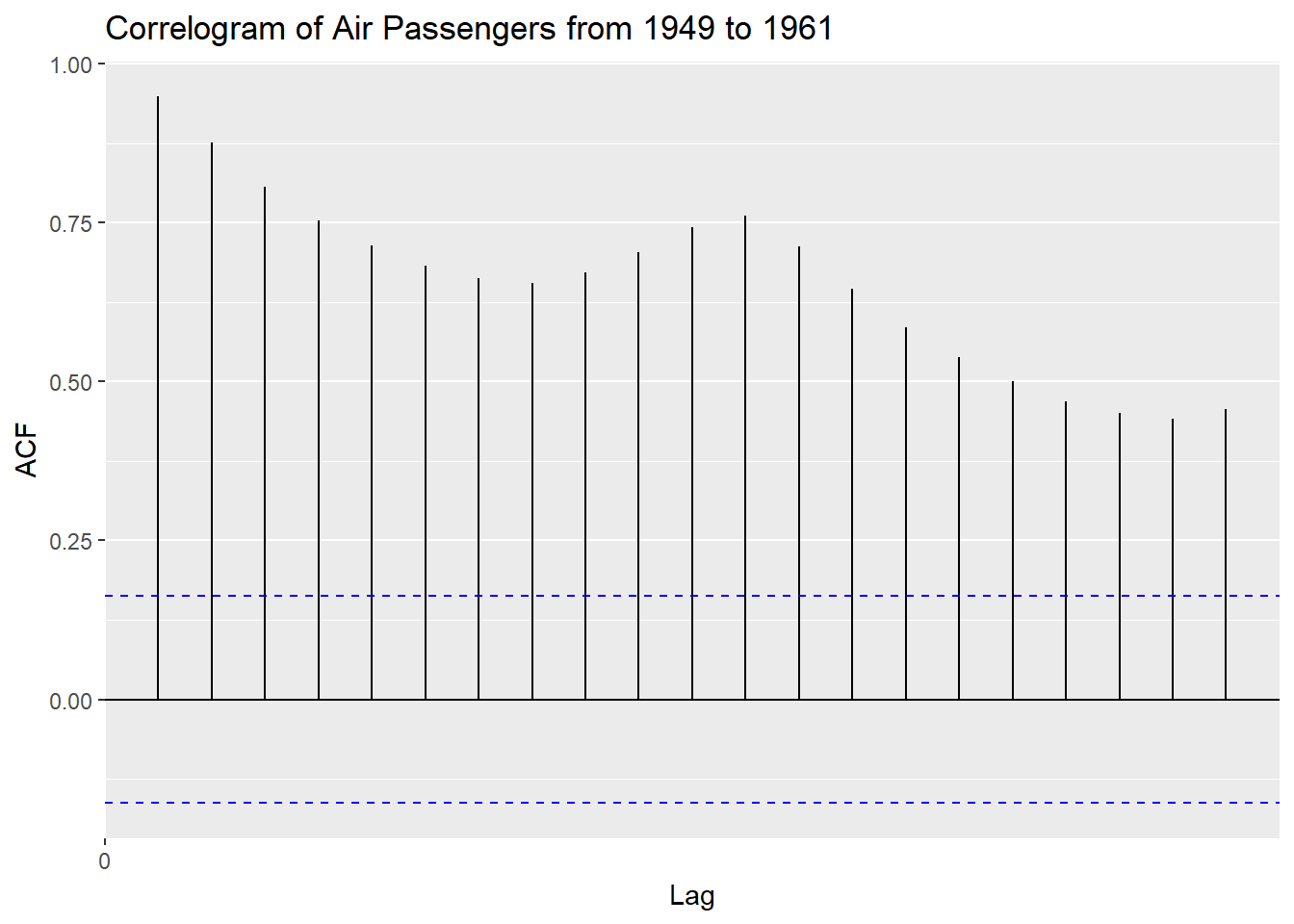The maximum at lag 1 or 12 months, indicates a positive relationship with the 12 month cycle.

Since we have already created the decomposeAP list object with a random component, we can plot the acf of the decomposeAP$random. # Review random time series for any missing values decomposeAP$random 
##            Jan       Feb       Mar       Apr       May       Jun       Jul
## 1949        NA        NA        NA        NA        NA        NA 0.9516643
## 1950 0.9626030 1.0714668 1.0374474 1.0140476 0.9269030 0.9650406 0.9835566
## 1951 1.0138446 1.0640180 1.0918541 1.0176651 1.0515825 0.9460444 0.9474041
## 1952 1.0258814 1.0939696 1.0134734 0.9695596 0.9632673 1.0003735 0.9468562
## 1953 0.9976684 1.0151646 1.0604644 1.0802327 1.0413329 0.9718056 0.9551933
## 1954 0.9829785 0.9232032 1.0044417 0.9943899 1.0119479 0.9978740 1.0237753
## 1955 1.0154046 0.9888241 0.9775844 1.0015732 0.9878755 1.0039635 1.0385512
## 1956 1.0066157 0.9970250 0.9876248 0.9968224 0.9985644 1.0275560 1.0217685
## 1957 0.9937293 0.9649918 0.9881769 0.9867637 0.9924177 1.0328601 1.0261250
## 1958 0.9954212 0.9522762 0.9469115 0.9383993 0.9715785 1.0261340 1.0483841
## 1959 0.9825176 0.9505736 0.9785278 0.9746440 1.0177637 0.9968613 1.0373136
## 1960 1.0039279 0.9590794 0.8940857 1.0064948 1.0173588 1.0120790        NA
##            Aug       Sep       Oct       Nov       Dec
## 1949 0.9534014 1.0022198 1.0040278 1.0062701 1.0118119
## 1950 0.9733720 1.0225047 0.9721928 0.9389527 1.0067914
## 1951 0.9397599 0.9888637 0.9938809 1.0235337 1.0250824
## 1952 0.9931171 0.9746302 1.0046687 1.0202797 1.0115407
## 1953 0.9894989 0.9934337 1.0192680 1.0009392 0.9915039
## 1954 0.9845184 0.9881036 0.9927613 0.9995143 0.9908692
## 1955 0.9831117 1.0032501 1.0003084 0.9827720 1.0125535
## 1956 1.0004765 1.0008730 0.9835071 0.9932761 0.9894251
## 1957 1.0312668 1.0236147 1.0108432 1.0212995 1.0005263
## 1958 1.0789695 0.9856540 0.9977971 0.9802940 0.9405687
## 1959 1.0531001 0.9974447 1.0013371 1.0134608 0.9999192
## 1960        NA        NA        NA        NA        NA
# Autoplot the random time series from 7:138 which exclude the NA values
autoplot(acf(decomposeAP\$random[7:138],plot=FALSE))+ labs(title="Correlogram of Air Passengers Random Component from 1949 to 1961")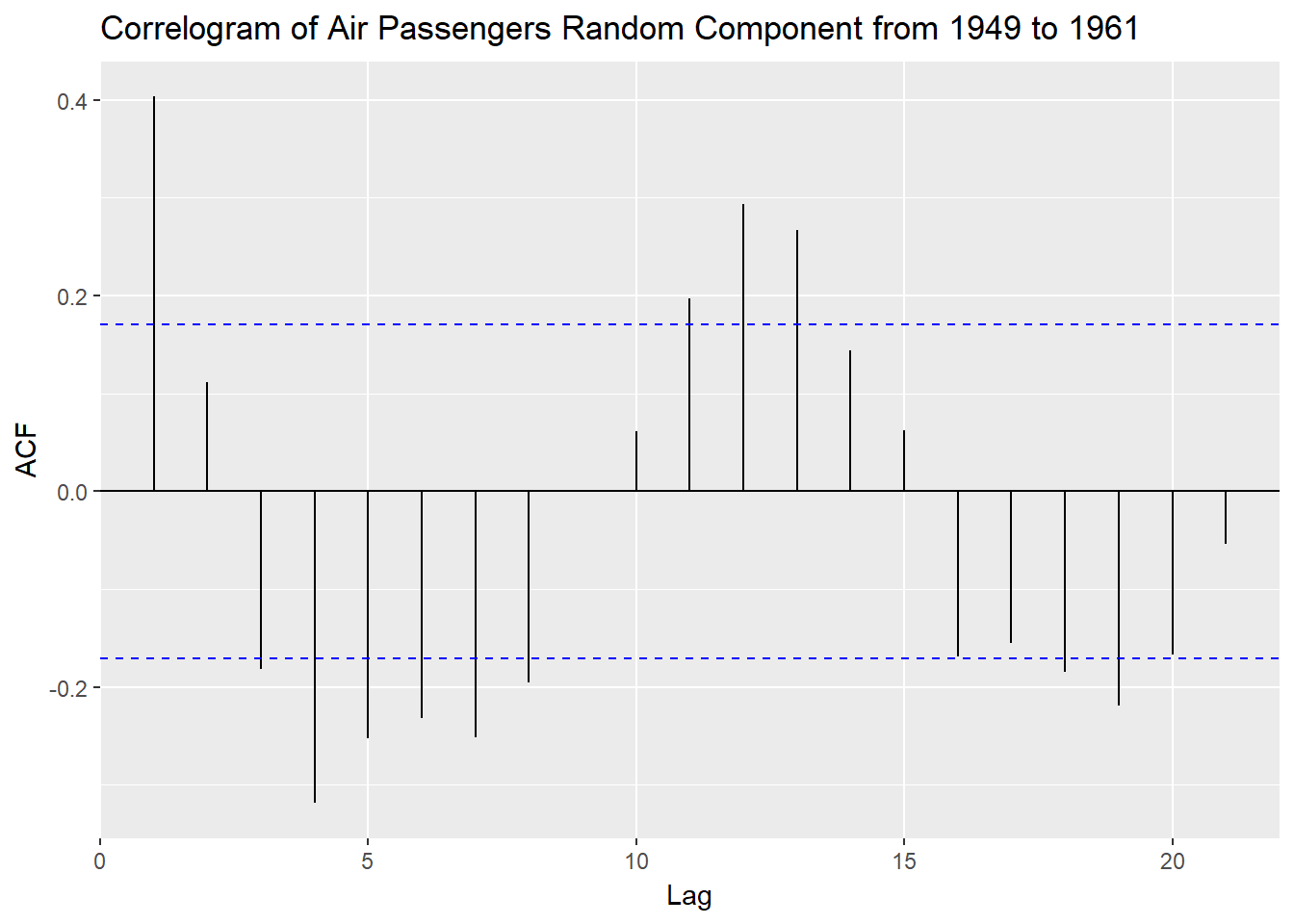We can see that the acf of the residuals is centered around 0.

## Part 4: FIT A TIME SERIES MODEL

1. Linear Model

Since there is an upwards trend we will look at a linear model first for comparison. We plot AirPassengers raw dataset with a blue linear model.

autoplot(AP) + geom_smooth(method="lm")+ labs(x ="Date", y = "Passenger numbers (1000's)", title="Air Passengers from 1949 to 1961")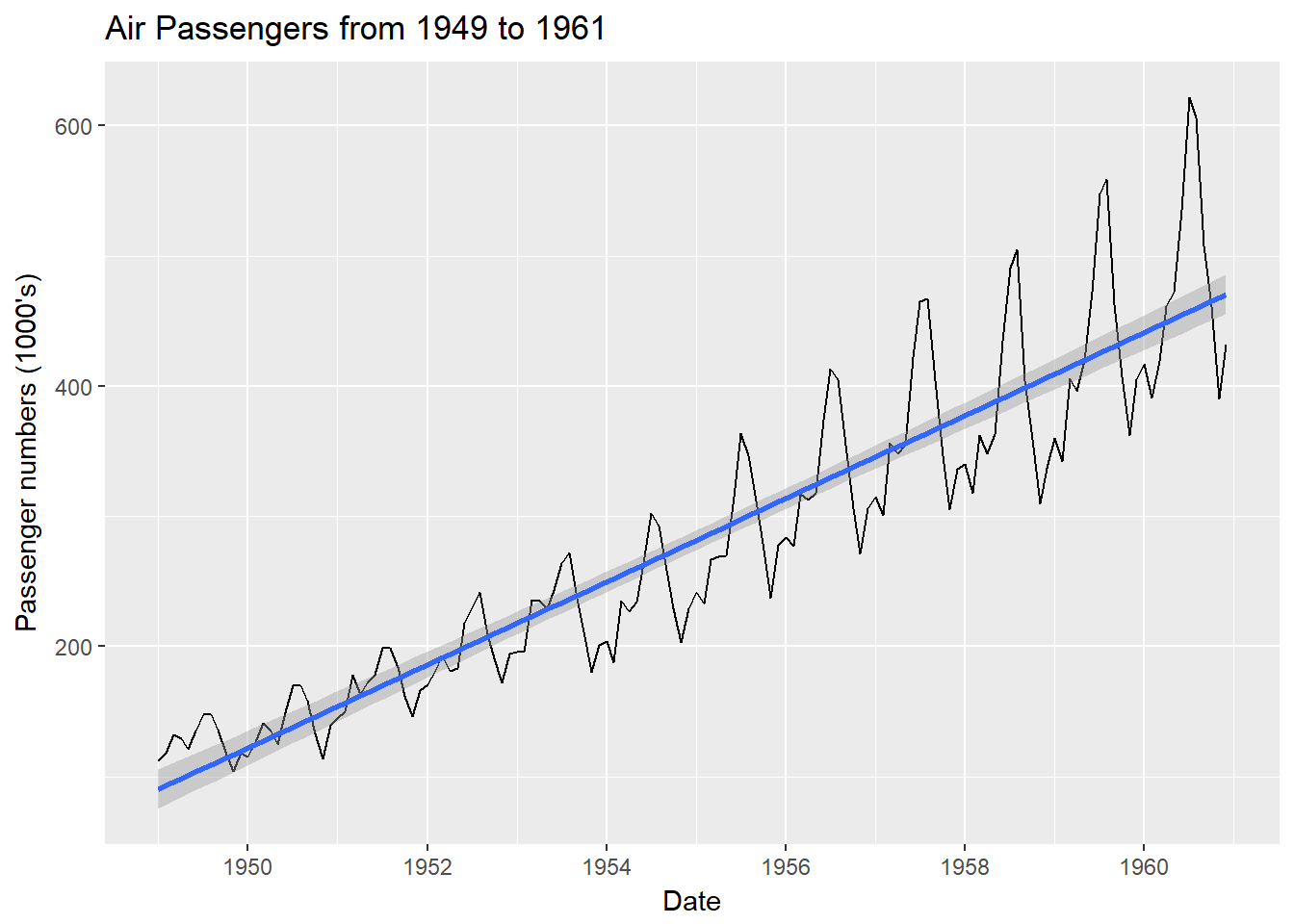This may not be the best model to fit as it doesn’t capture the seasonality and multiplicative effects over time.

2. ARIMA Model

Use the auto.arima function from the forecast R package to fit the best model and coefficients, given the default parameters including seasonality as TRUE. Note we have used the ARIMA modeling procedure as referenced

arimaAP <- auto.arima(AP)
arimaAP
## Series: AP
## ARIMA(2,1,1)(0,1,0)
##
## Coefficients:
##          ar1     ar2      ma1
##       0.5960  0.2143  -0.9819
## s.e.  0.0888  0.0880   0.0292
##
## sigma^2 estimated as 132.3:  log likelihood=-504.92
## AIC=1017.85   AICc=1018.17   BIC=1029.35

The ARIMA(2,1,1)(0,1,0) model parameters are lag 1 differencing (d), an autoregressive term of second lag (p) and a moving average model of order 1 (q). Then the seasonal model has an autoregressive term of first lag (D) at model period 12 units, in this case months.

The ARIMA fitted model is:

$\hat{Y} = 0.5960Y_{t-2} +0.2143Y_{t-12} - 0.9819 e_{t-1} + E$

where E is some error.

The ggtsdiag function from ggfortify R package performs model diagnostics of the residuals and the acf. will include a autocovariance plot.

ggtsdiag(arimaAP)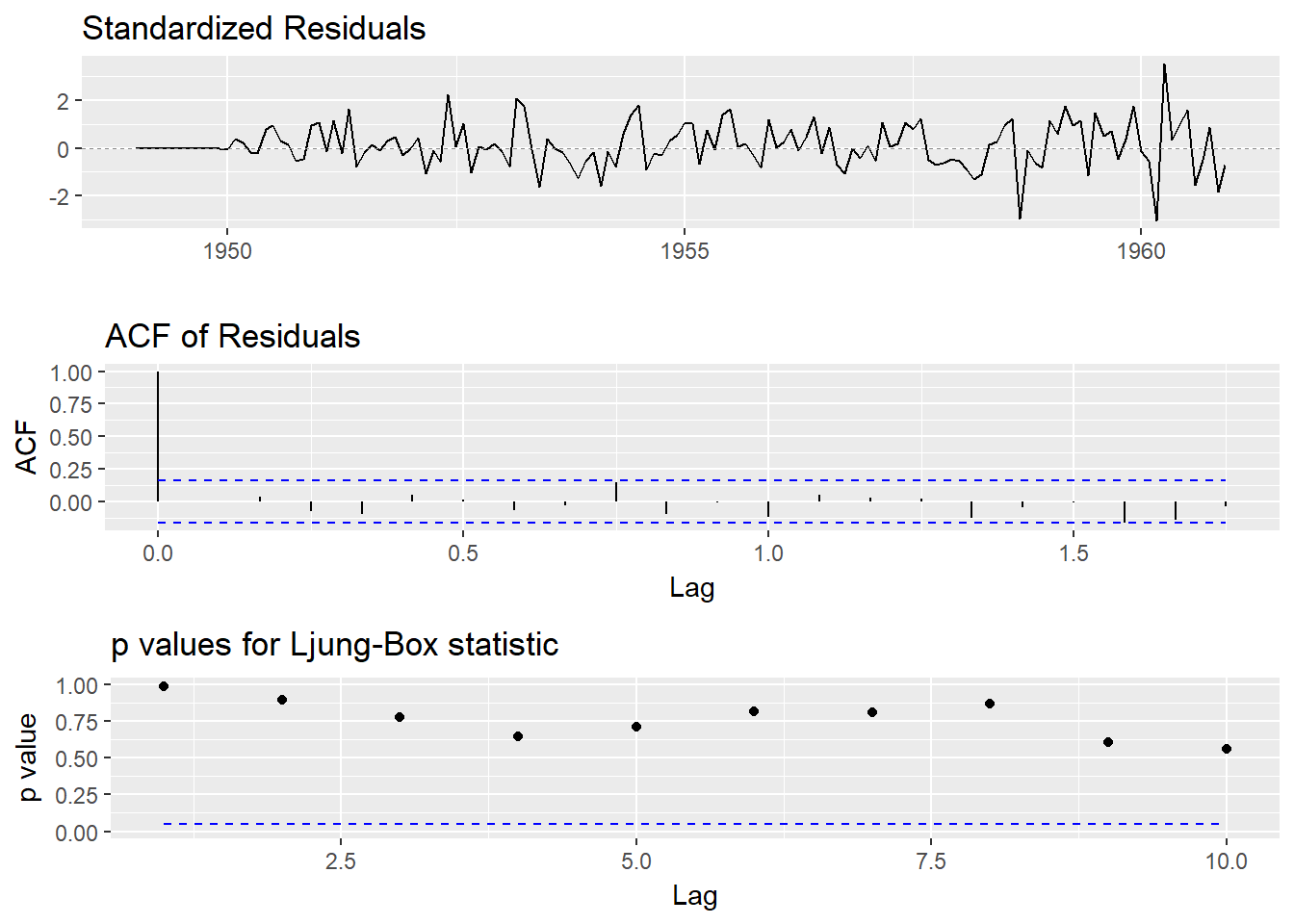The residual plots appear to be centered around 0 as noise, with no pattern. the arima model is a fairly good fit.

## Part 5: CALCULATE FORECASTS

Finally we can plot a forecast of the time series using the forecast function, again from the forecast R package, with a 95% confidence interval where h is the forecast horizon periods in months.

forecastAP <- forecast(arimaAP, level = c(95), h = 36)
autoplot(forecastAP)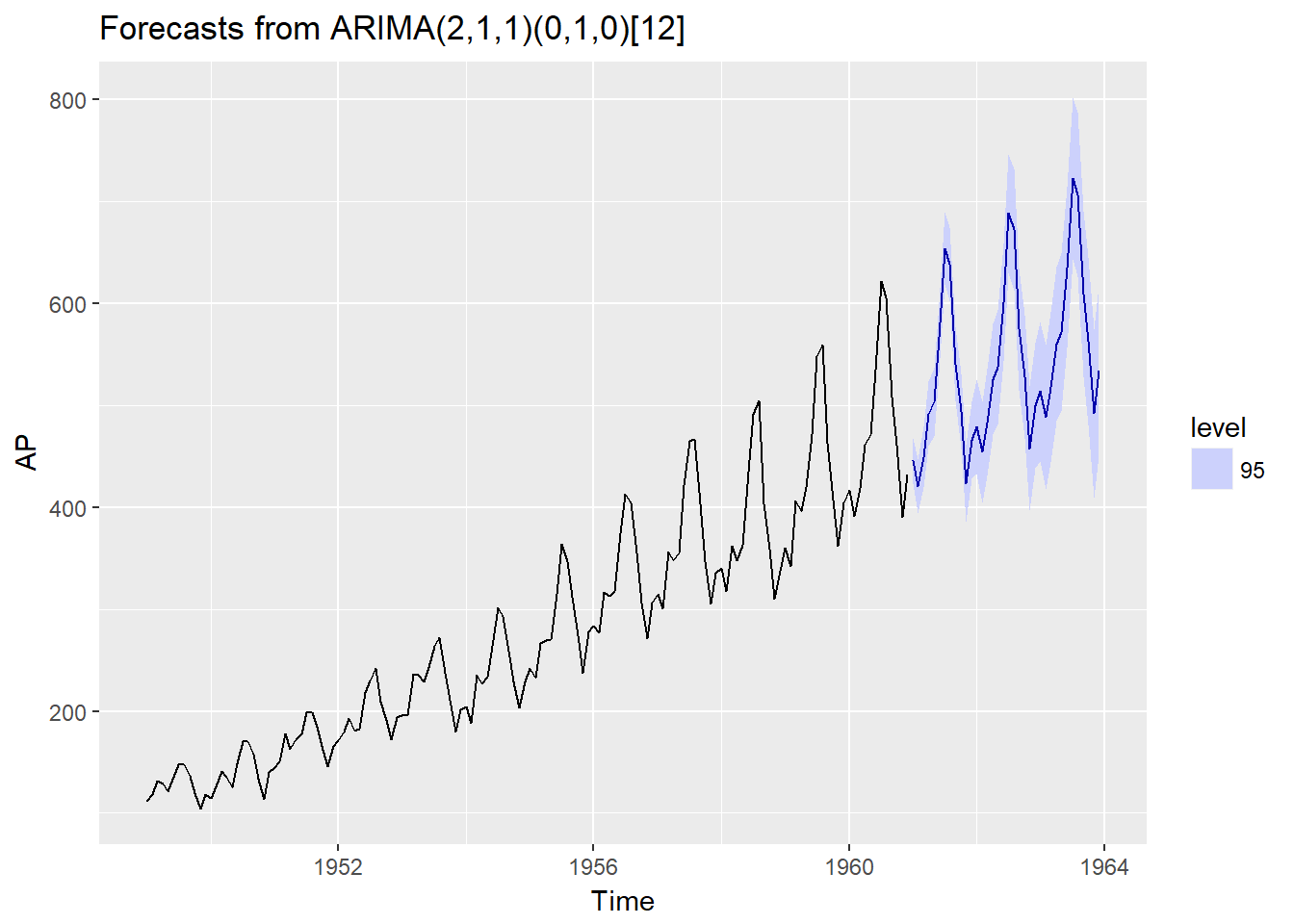To summarize, this has been an exercise in ARIMA modeling and using time series R packages ggfortify, tseries and forecast. It is a good basis to move on to more complicated time series datasets, models and comparisons in R.